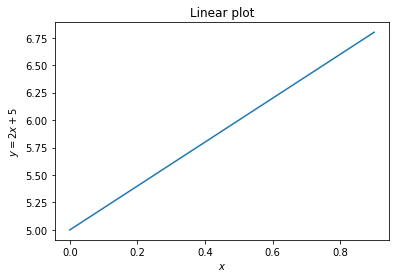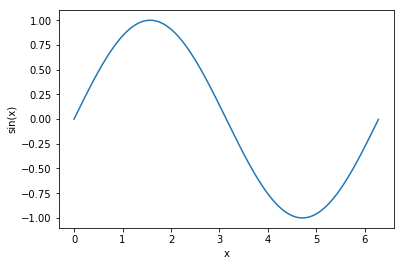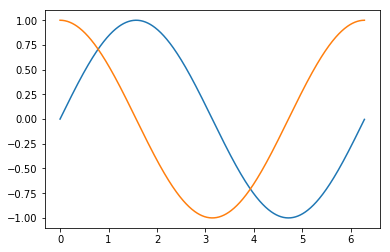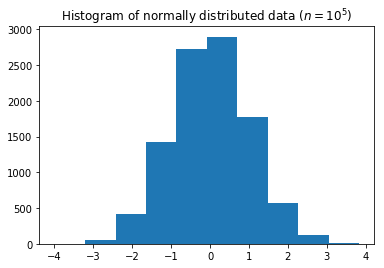# Numerical Computing in Python¶

(c) 2019 Steve Phelps

## Overview¶

• Floating-point representation
• Arrays and Matrices with numpy
• Basic plotting with matplotlib
• Pseudo-random variates with numpy.random

## Representing continuous values¶

• Digital computers are inherently discrete.

• Real numbers $x \in R$ cannot always be represented exactly in a digital computer.

• They are stored in a format called floating-point.

• IEEE Standard 754 specifies a universal format across different implementations.

• As always there are deviations from the standard.
• There are two standard sizes of floating-point numbers: 32-bit and 64-bit.

• 64-bit numbers are called double precision, are sometimes called double values.

• IEEE floating-point calculations are performed in hardware on modern computers.

• How can we represent aribitrary real values using only 32 bits?

## Fixed-point verses floating-point¶

• One way we could discretise continuous values is to represent them as two integers $x$ and $y$.

• The final value is obtained by e.g. $r = x + y \times 10^{-5}$.

• So the number $500.4421$ would be represented as the tuple $x = 500$, $y = 44210$.

• The exponent $5$ is fixed for all computations.

• This number represents the precision with which we can represent real values.

• It corresponds to the where we place we place the decimal point.

• This scheme is called fixed precision.

• It is useful in certain circumstances, but suffers from many problems, in particular it can only represent a very limited range of values.

• In practice, we use variable precision, also known as floating point.

## Scientific Notation¶

• Humans also use a form of floating-point representation.

• In Scientific notation, all numbers are written in the form $m \times 10^n$.

• When represented in ASCII, we abbreviate this <m>e<n>, for example 6.72e+11 = $6.72 \times 10^{11}$.

• The integer $m$ is called the significand or mantissa.

• The integer $n$ is called the exponent.

• The integer $10$ is the base.

## Scientific Notation in Python¶

• Python uses Scientific notation when it displays floating-point numbers:
In :
print(672000000000000000.0)

6.72e+17

• Note that internally, the value is not represented exactly like this.

• Scientific notation is a convention for writing or rendering numbers, not representing them digitally.

## Floating-point representation¶

• Floating point numbers use a base of $2$ instead of $10$.

• Additionally, the mantissa are exponent are stored in binary.

• Therefore we represent floating-point numbers as $m \times 2 ^ e$.

• The integers $m$ (mantissa) and $e$ (exponent) are stored in binary.

• The mantissa uses two's complement to represent positive and negative numbers.

• One bit is reserved as the sign-bit: 1 for negative, 0 for positive values.
• The mantissa is normalised, so we assume that it starts with the digit $1$ (which is not stored).

## Bias¶

• We also need to represent signed exponents.

• The exponent does not use two's complement.

• Instead a bias value is subtracted from the stored exponent ($s$) to obtain the final value ($e$).

• Double-precision values use a bias of $b = 1023$, and single-precision uses a bias value of $b = 127$.

• The actual exponent is given by $e = s - b$ where $s$ is the stored exponent.

• The stored exponent values $s=0$ and $s=1024$ are reserved for special values- discussed later.

• The stored exponent $s$ is represented in binary without using a sign bit.

## Double and single precision formats¶

The number of bits allocated to represent each integer component of a float is given below:

Format Sign Exponent Mantissa Total
single 1 8 23 32
double 1 11 52 64

## Loss of precision¶

• We cannot represent every value in floating-point.

• Consider single-precision (32-bit).

• Let's try to represent $4,039,944,879$.

## Loss of precision¶

• As a binary integer we write $4,039,944,879$ as:

11110000 11001100 10101010 10101111

• This already takes up 32-bits.

• The mantissa only allows us to store 24-bit integers.

• So we have to round. We store it as:

+1.1110000 11001100 10101011e+31

• Which gives us

+11110000 11001100 10101011 0000000

$= 4,039,944,960$

## Ranges of floating-point values¶

In single precision arithmetic, we cannot represent the following values:

• Negative numbers less than $-(2-2^{-23}) \times 2^{127}$

• Negative numbers greater than $-2^{-149}$

• Positive numbers less than $2^{-149}$

• Positive numbers greater than $(2-2^{-23}) \times 2^{127}$

Attempting to represent these numbers results in overflow or underflow.

## Effective floating-point range¶

Format Binary Decimal
single $\pm (2-2^{-23}) \times 2^{127}$ $\approx \pm 10^{38.53}$
double $\pm (2-2^{-52}) \times 2^{1023}$ $\approx \pm 10^{308.25}$
In :
import sys
sys.float_info.max

Out:
1.7976931348623157e+308
In :
sys.float_info.min

Out:
2.2250738585072014e-308
In :
sys.float_info

Out:
sys.float_info(max=1.7976931348623157e+308, max_exp=1024, max_10_exp=308, min=2.2250738585072014e-308, min_exp=-1021, min_10_exp=-307, dig=15, mant_dig=53, epsilon=2.220446049250313e-16, radix=2, rounds=1)

## Range versus precision¶

• With a fixed number of bits, we have to choose between:
• maximising the range of values (minimum to maximum) we can represent,
• maximising the precision with-which we can represent each indivdual value.
• These are conflicting objectives:

• we can increase range, but only by losing precision,
• we can increase precision, but only by decreasing range.
• Floating-point addresses this dilemma by allowing the precision to vary ("float") according to the magnitude of the number we are trying to represent.

## Floating-point density¶

• Floating-point numbers are unevenly-spaced over the line of real-numbers.

• The precision decreases as we increase the magnitude.## Representing Zero¶

• Zero cannot be represented straightforwardly because we assume that all mantissa values start with the digit 1.

• Zero is stored as a special-case, by setting mantissa and exponent both to zero.

• The sign-bit can either be set or unset, so there are distinct positive and negative representations of zero.

## Zero in Python¶

In :
x = +0.0
x

Out:
0.0
In :
y = -0.0
y

Out:
-0.0
• However, these are considered equal:
In :
x == y

Out:
True

## Infinity¶

• Positive overflow results in a special value of infinity (in Python inf).

• This is stored with an exponent consiting of all 1s, and a mantissa of all 0s.

• The sign-bit allows us to differentiate between negative and positive overflow: $-\infty$ and $+\infty$.

• This allows us to carry on calculating past an overflow event.

## Infinity in Python¶

In :
x = 1e300 * 1e100
x

Out:
inf
In :
x = x + 1
x

Out:
inf

## Negative infinity in Python¶

In :
x > 0

Out:
True
In :
y = -x
y

Out:
-inf
In :
y < x

Out:
True

## Not A Number (NaN)¶

• Some mathematical operations on real numbers do not map onto real numbers.

• These results are represented using the special value to NaN which represents "not a (real) number".

• NaN is represented by an exponent of all 1s, and a non-zero mantissa.

## NaN in Python¶

In :
from numpy import sqrt, inf, isnan, nan
x = sqrt(-1)
x

/home/sphelps/anaconda3/lib/python3.6/site-packages/ipykernel_launcher.py:2: RuntimeWarning: invalid value encountered in sqrt


Out:
nan
In :
y = inf - inf
y

Out:
nan

## Comparing nan values in Python¶

• Beware of comparing nan values
In :
x == y

Out:
False
• To test whether a value is nan use the isnan function:
In :
isnan(x)

Out:
True

## NaN is not the same as None¶

• None represents a missing value.

• NaN represents an invalid floating-point value.

• These are fundamentally different entities:

In :
nan is None

Out:
False
In :
isnan(None)

---------------------------------------------------------------------------
TypeError                                 Traceback (most recent call last)
<ipython-input-20-4a0142ec4134> in <module>()
----> 1 isnan(None)

TypeError: ufunc 'isnan' not supported for the input types, and the inputs could not be safely coerced to any supported types according to the casting rule ''safe''

## Beware finite precision¶

In :
x = 0.1 + 0.2

In :
x == 0.3

Out:
False
In :
x

Out:
0.30000000000000004

## Relative and absolute error¶

• Consider a floating point number $x_{fp}$ which represents a real number $x \in \mathbf{R}$.

• In general, we cannot precisely represent the real number; that is $x_{fp} \neq x$.

• The absolute error $r$ is $r = x - x_{fp}$.

• The relative error $R$ is:

\begin{equation} R = \frac{x - x_{fp}}{x} \end{equation}

## Numerical Methods¶

• In e.g. simulation models or quantiative analysis we typically repeatedly update numerical values inside long loops.

• Programs such as these implement numerical algorithms.

• It is very easy to introduce bugs into code like this.

## Numerical stability¶

• The round-off error associated with a result can be compounded in a loop.

• If the error increases as we go round the loop, we say the algorithm is numerically unstable.

• Mathematicians design numerically stable algorithms using numerical analysis.

## Catastrophic Cancellation¶

• Suppose we have two real values $x$, and $y = x + \epsilon$.

• $\epsilon$ is very small and $x$ is very large.

• $x$ has an exact floating point representation

• However, because of lack of precision $x$ and $y$ have the same floating point representation.

• i.e. they are represented as the same sequence of 64-bits
• Consider wheat happens when we compute $y - x$ in floating-point.

## Catestrophic Cancellation and Relative Error¶

• Catestrophic cancellation results in very large relative error.

• If we calculate $y - x$ in floating-point we will obtain the result 0.

• The correct value is $(x + \epsilon) - x = \epsilon$.

• The relative error is

\begin{equation} \frac {\epsilon - 0} {\epsilon} = 1 \end{equation}
• That is, the relative error is $100\%$.

• This can result in catastrophy.

## Catastrophic Cancellation in Python¶

In :
x = 3.141592653589793
x

Out:
3.141592653589793
In :
y = 6.022e23
x = (x + y) - y

In :
x

Out:
0.0

• Addition, on the other hand, is not catestrophic.
In :
z = x + y
z

Out:
6.022e+23
• The above result is still inaccurate with an absolute error $r \approx \pi$.

• However, let's examine the relative error:

\begin{equation} R = \frac {1.2044 \times 10^{24} - (1.2044 \times 10^{24} + \pi)} {1.2044 \times 10^{24} + \pi} \\ \approx 10^{-24} \end{equation}
• Here we see that that the relative error from the addition is miniscule compared with the cancellation.

### Floating-point arithemetic is nearly always inaccurate.¶

• You can hardly-ever eliminate absolute rounding error when using floating-point.

• The best we can do is to take steps to minimise error, and prevent it from increasing as your calculation progresses.

• Cancellation can be catastrophic, because it can greatly increase the relative error in your calculation.

## Use a well-tested library for numerical algorithms.¶

• Avoid subtracting two nearly-equal numbers.

• Especially in a loop!

• Better-yet use a well-validated existing implementation in the form of a numerical library.

## Importing numpy¶

• Functions for numerical computiing are provided by a separate module called numpy.

• Before we use the numpy module we must import it.

• By convention, we import numpy using the alias np.

• Once we have done this we can prefix the functions in the numpy library using the prefix np.

In :
import numpy as np

• We can now use the functions defined in this package by prefixing them with np.

## Arrays¶

• Arrays represent a collection of values.

• In contrast to lists:

• arrays typically have a fixed length
• they can be resized, but this involves an expensive copying process.
• and all values in the array are of the same type.
• typically we store floating-point values.
• Like lists:

• arrays are mutable;
• we can change the elements of an existing array.

## Arrays in numpy¶

• Arrays are provided by the numpy module.

• The function array() creates an array given a list.

In :
import numpy as np
x = np.array([0, 1, 2, 3, 4])
x

Out:
array([0, 1, 2, 3, 4])

## Array indexing¶

• We can index an array just like a list
In :
x

Out:
4
In :
x = 2
x

Out:
array([0, 1, 2, 3, 2])

## Arrays are not lists¶

• Although this looks a bit like a list of numbers, it is a fundamentally different type of value:
In :
type(x)

Out:
numpy.ndarray
• For example, we cannot append to the array:
In :
x.append(5)

---------------------------------------------------------------------------
AttributeError                            Traceback (most recent call last)
<ipython-input-32-7e52d4acf950> in <module>()
----> 1 x.append(5)

AttributeError: 'numpy.ndarray' object has no attribute 'append'

## Populating Arrays¶

• To populate an array with a range of values we use the np.arange() function:
In :
x = np.arange(0, 10)
print(x)

[0 1 2 3 4 5 6 7 8 9]

• We can also use floating point increments.
In :
x = np.arange(0, 1, 0.1)
print(x)

[0.  0.1 0.2 0.3 0.4 0.5 0.6 0.7 0.8 0.9]


## Functions over arrays¶

• When we use arithmetic operators on arrays, we create a new array with the result of applying the operator to each element.
In :
y = x * 2
y

Out:
array([0. , 0.2, 0.4, 0.6, 0.8, 1. , 1.2, 1.4, 1.6, 1.8])
• The same goes for numerical functions:
In :
x = np.array([-1, 2, 3, -4])
y = abs(x)
y

Out:
array([1, 2, 3, 4])

## Vectorized functions¶

• Note that not every function automatically works with arrays.

• Functions that have been written to work with arrays of numbers are called vectorized functions.

• Most of the functions in numpy are already vectorized.

• You can create a vectorized version of any other function using the higher-order function numpy.vectorize().

## vectorize example¶

In :
def myfunc(x):
if x >= 0.5:
return x
else:
return 0.0

fv = np.vectorize(myfunc)

In :
x = np.arange(0, 1, 0.1)
x

Out:
array([0. , 0.1, 0.2, 0.3, 0.4, 0.5, 0.6, 0.7, 0.8, 0.9])
In :
fv(x)

Out:
array([0. , 0. , 0. , 0. , 0. , 0.5, 0.6, 0.7, 0.8, 0.9])

## Testing for equality¶

• Because of finite precision we need to take great care when comparing floating-point values.

• The numpy function allclose() can be used to test equality of floating-point numbers within a relative tolerance.

• It is a vectorized function so it will work with arrays as well as single floating-point values.

In :
x = 0.1 + 0.2
y = 0.3
x == y

Out:
False
In :
np.allclose(x, y)

Out:
True

## Plotting with matplotlib¶

• We will use a module called matplotlib to plot some simple graphs.

• This module has a nested module called pyplot.

• By convention we import this with the alias plt.

• This module provides functions which are very similar to MATLAB plotting commands.

In [ ]:
import matplotlib.pyplot as plt


### A simple linear plot¶

In :
x = np.arange(0, 1, 0.1)
y = x*2 + 5
plt.plot(x, y)
plt.xlabel('$x$')
plt.ylabel('$y = 2x + 5$')
plt.title('Linear plot')
plt.show()### Plotting a sine curve¶

In :
from numpy import pi, sin

x = np.arange(0, 2*pi, 0.01)
y = sin(x)
plt.plot(x, y)
plt.xlabel('x')
plt.ylabel('sin(x)')
plt.show()## Multi-dimensional data¶

• Numpy arrays can hold multi-dimensional data.

• To create a multi-dimensional array, we can pass a list of lists to the array() function:

In :
import numpy as np

x = np.array([[1,2], [3,4]])
x

Out:
array([[1, 2],
[3, 4]])

### Arrays containing arrays¶

• A multi-dimensional array is an array of an arrays.

• The outer array holds the rows.

• Each row is itself an array:

In :
x

Out:
array([1, 2])
In :
x

Out:
array([3, 4])
• So the element in the second row, and first column is:
In :
x

Out:
3

### Matrices¶

• We can create a matrix from a multi-dimensional array.
In :
M = np.matrix(x)
M

Out:
matrix([[1, 2],
[3, 4]])

### Plotting multi-dimensional with matrices¶

• If we supply a matrix to plot() then it will plot the y-values taken from the columns of the matrix (notice the transpose in the example below).
In :
from numpy import pi, sin, cos

x = np.arange(0, 2*pi, 0.01)
y = sin(x)
ax = plt.plot(x, np.matrix([sin(x), cos(x)]).T)
plt.show()### Performance¶

• When we use numpy matrices in Python the corresponding functions are linked with libraries written in C and FORTRAN.

• For example, see the BLAS (Basic Linear Algebra Subprograms) library.

• These libraries are very fast.

• Vectorised code can be more easily ported to frameworks like TensorFlow so that operations are performed in parallel using GPU hardware.

### Matrix Operators¶

• Once we have a matrix, we can perform matrix computations.

• To compute the transpose and inverse use the T and I attributes:

To compute the transpose $M^{T}$

In :
M.T

Out:
matrix([[1, 3],
[2, 4]])

To compute the inverse $M^{-1}$

In :
M.I

Out:
matrix([[-2. ,  1. ],
[ 1.5, -0.5]])

### Matrix Dimensions¶

• The total number of elements, and the dimensions of the array:
In :
M.size

Out:
4
In :
M.shape

Out:
(2, 2)
In :
len(M.shape)

Out:
2

### Creating Matrices from strings¶

• We can also create arrays directly from strings, which saves some typing:
In :
I2 = np.matrix('2 0; 0 2')
I2

Out:
matrix([[2, 0],
[0, 2]])
• The semicolon starts a new row.

### Matrix Multiplication¶

Now that we have two matrices, we can perform matrix multiplication:

In :
M * I2

Out:
matrix([[2, 4],
[6, 8]])

### Matrix Indexing¶

In :
M[:,1]

Out:
matrix([,
])

### Slices are references¶

• If we use this is an assignment, we create a reference to the sliced elements, not a copy.
In :
V = M[:,1]  # This does not make a copy of the elements!
V

Out:
matrix([,
])
In :
M[0,1] = -2
V

Out:
matrix([[-2],
[ 4]])

### Copying matrices and vectors¶

• To copy a matrix, or a slice of its elements, use the function np.copy():
In :
M = np.matrix('1 2; 3 4')
V = np.copy(M[:,1])  # This does copy the elements.
V

Out:
array([,
])
In :
M[0,1] = -2
V

Out:
array([,
])

## Sums¶

One way we could sum a vector or matrix is to use a for loop.

In :
vector = np.arange(0.0, 100.0, 10.0)
vector

Out:
array([ 0., 10., 20., 30., 40., 50., 60., 70., 80., 90.])
In :
result = 0.0
for x in vector:
result = result + x
result

Out:
450.0
• This is not the most efficient way to compute a sum.

## Efficient sums¶

• Instead of using a for loop, we can use a numpy function sum().

• This function is written in the C language, and is very fast.

In :
vector = np.array([0, 1, 2, 3, 4])
print(np.sum(vector))

10


## Summing rows and columns¶

• When dealing with multi-dimensional data, the 'sum()' function has a named-argument axis which allows us to specify whether to sum along, each rows or columns.
In :
matrix = np.matrix('1 2 3; 4 5 6; 7 8 9')
print(matrix)

[[1 2 3]
[4 5 6]
[7 8 9]]


### To sum along rows:¶

In :
np.sum(matrix, axis=0)

Out:
matrix([[12, 15, 18]])

### To sum along columns:¶

In :
np.sum(matrix, axis=1)

Out:
matrix([[ 6],
,
])

## Cumulative sums¶

• Suppose we want to compute $y_n = \sum_{i=1}^{n} x_i$ where $\vec{x}$ is a vector.
In :
import numpy as np
x = np.array([0, 1, 2, 3, 4])
y = np.cumsum(x)
print(y)

[ 0  1  3  6 10]


## Cumulative sums along rows and columns¶

In :
x = np.matrix('1 2 3; 4 5 6; 7 8 9')
print(x)

[[1 2 3]
[4 5 6]
[7 8 9]]

In :
y = np.cumsum(x)
np.cumsum(x, axis=0)

Out:
matrix([[ 1,  2,  3],
[ 5,  7,  9],
[12, 15, 18]])
In :
np.cumsum(x, axis=1)

Out:
matrix([[ 1,  3,  6],
[ 4,  9, 15],
[ 7, 15, 24]])

## Cumulative products¶

• Similarly we can compute $y_n = \Pi_{i=1}^{n} x_i$ using cumprod():
In :
import numpy as np
x = np.array([1, 2, 3, 4, 5])
np.cumprod(x)

Out:
array([  1,   2,   6,  24, 120])
• We can compute cummulative products along rows and columns using the axis parameter, just as with the cumsum() example.

## Generating (pseudo) random numbers¶

• The nested module numpy.random contains functions for generating random numbers from different probability distributions.
In :
from numpy.random import normal, uniform, exponential, randint

• Suppose that we have a random variable $\epsilon \sim N(0, 1)$.

• In Python we can draw from this distribution like so:

In :
epsilon = normal()
epsilon

Out:
0.1465312427787133
• If we execute another call to the function, we will make a new draw from the distribution:
In :
epsilon = normal()
epsilon

Out:
-2.3135050810408773

## Pseudo-random numbers¶

• Strictly speaking, these are not random numbers.

• They rely on an initial state value called the seed.

• If we know the seed, then we can predict with total accuracy the rest of the sequence, given any "random" number.

• Nevertheless, statistically they behave like independently and identically-distributed values.

• Statistical tests for correlation and auto-correlation give insignificant results.
• For this reason they called pseudo-random numbers.

• The algorithms for generating them are called Pseudo-Random Number Generators (PRNGs).

• Some applications, such as cryptography, require genuinely unpredictable sequences.

• never use a standard PRNG for these applications!

## Managing seed values¶

• In some applications we need to reliably reproduce the same sequence of pseudo-random numbers that were used.

• We can specify the seed value at the beginning of execution to achieve this.

• Use the function seed() in the numpy.random module.

## Setting the seed¶

In :
from numpy.random import seed

seed(5)

In :
normal()

Out:
0.44122748688504143
In :
normal()

Out:
-0.33087015189408764
In :
seed(5)

In :
normal()

Out:
0.44122748688504143
In :
normal()

Out:
-0.33087015189408764

## Drawing multiple variates¶

• To generate more than number, we can specify the size parameter:
In :
normal(size=10)

Out:
array([ 2.43077119, -0.25209213,  0.10960984,  1.58248112, -0.9092324 ,
-0.59163666,  0.18760323, -0.32986996, -1.19276461, -0.20487651])
• If you are generating very many variates, this will be much faster than using a for loop
• We can also specify more than one dimension:
In :
normal(size=(5,5))

Out:
array([[-0.35882895,  0.6034716 , -1.66478853, -0.70017904,  1.15139101],
[ 1.85733101, -1.51117956,  0.64484751, -0.98060789, -0.85685315],
[-0.87187918, -0.42250793,  0.99643983,  0.71242127,  0.05914424],
[-0.36331088,  0.00328884, -0.10593044,  0.79305332, -0.63157163],
[-0.00619491, -0.10106761, -0.05230815,  0.24921766,  0.19766009]])

## Histograms¶

• We can plot a histograms of randomly-distributed data using the hist() function from matplotlib:
In :
import matplotlib.pyplot as plt

data = normal(size=10000)
ax = plt.hist(data)
plt.title('Histogram of normally distributed data ($n=10^5$)')
plt.show()## Computing histograms as matrices¶

• The function histogram() in the numpy module will count frequencies into bins and return the result as a 2-dimensional array.
In :
import numpy as np
np.histogram(data)

Out:
(array([  23,  136,  618, 1597, 2626, 2635, 1620,  599,  130,   16]),
array([-3.59780883, -2.87679609, -2.15578336, -1.43477063, -0.71375789,
0.00725484,  0.72826758,  1.44928031,  2.17029304,  2.89130578,
3.61231851]))

## Descriptive statistics¶

• We can compute the descriptive statistics of a sample of values using the numpy functions mean() and var() to compute the sample mean $\bar{X}$ and sample variance $\sigma_{X}^2$ .
In :
np.mean(data)

Out:
-0.00045461080333497925
In :
np.var(data)

Out:
1.0016048722546331
• These functions also have an axis parameter to compute mean and variances of columns or rows of a multi-dimensional data-set.

## Descriptive statistics with nan values¶

• If the data contains nan values, then the descriptive statistics will also be nan.
In :
from numpy import nan
import numpy as np
data = np.array([1, 2, 3, 4, nan])
np.mean(data)

Out:
nan
• To omit nan values from the calculation, use the functions nanmean() and nanvar():
In :
np.nanmean(data)

Out:
2.5

## Discrete random numbers¶

• The randint() function in numpy.random can be used to draw from a uniform discrete probability distribution.

• It takes two parameters: the low value (inclusive), and the high value (exclusive).

• So to simulate one roll of a die, we would use the following Python code.

In :
die_roll = randint(0, 6) + 1
die_roll

Out:
4
• Just as with the normal() function, we can generate an entire sequence of values.

• To simulate a Bernoulli process with $n=20$ trials:

In :
bernoulli_trials = randint(0, 2, size = 20)
bernoulli_trials

Out:
array([1, 1, 0, 1, 1, 1, 0, 1, 0, 0, 1, 1, 1, 0, 0, 0, 0, 1, 1, 0])

Acknowledgements

The early sections of this notebook were adapted from an online article by Steve Hollasch.